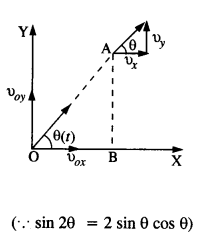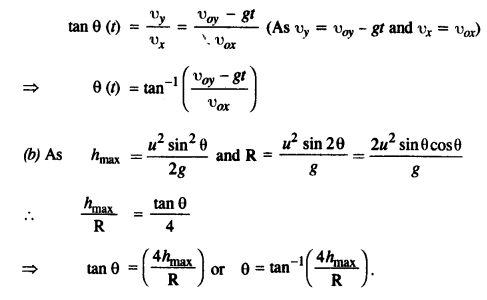## NCERT Solutions for Class 11 Physics Chapter 4 Motion in a Plane

These Solutions are part of NCERT Solutions for Class 11 Physics. Here we have given NCERT Solutions for Class 11 Physics Chapter 4 Motion in a Plane

Question 1.
State, for each of the following physical quantities, if it is a scalar or a vector :
Volume, mass, speed, acceleration, density, number of moles, velocity, angular frequency, displacement, angular velocity.Average Velocity Calculator – Calculate Average Velocity.

Question 2.
Pick out the two scalar quantities in the following list:
Force, Angular momentum, work, current, linear momentum, electric field, average velocity, magnetic moment, reaction as per Newton’s third law, relative velocity.
Work and Current.

Question 3.
Pick out the only vector quantity in the following list:
Temperature, pressure, impulse, time, power, total path length, energy, gravitational potential, coefficient of friction, charge.
Impulse.

Question 4.
State with reasons, whether the following algebraic operations with scalar and vector physical quantities are meaningful:

2. adding a scalar to a vector of the same dimensions,
3. multiplying any vector by any scalar,
4. multiplying any two scalars,
6. adding a component of a vector to the same vector.

1. Not meaningful. Two scalars of different dimensions/units cannot be added.
2. Not meaningful. A scalar and a vector cannot be added.
3. Meaningful. A scalar and vector can be multiplied irrespective of their dimensions/units.
4. Meaningful. Two scalars can be multiplied irrespective of their dimensions/units.
5. eNot meaningful. Two vectors of different dimensions/units cannot be added.
6. Not meaningful. A component of a vector cannot be added to it.

Question 5.
Read each statement below carefully and state with reasons, if it is true or false :
(a) The magnitude of a vector is always a scalar,
(b) each component of a vector is always a scalar,
(c) the total path length is always equal to the magnitude of the displacement vector of a particle
(d) the average speed of a particle (defined as total path length divided by the time taken to cover the path is either greater or equal to the magnitude of average velocity of the particle over the same interval of time,
(e) Three vectors not lying in a plane can never add up to give a null vector.
(a) True, magnitude of the velocity of a body moving in a straight line may be equal to the speed of the body.
(b) False, each component of a vector is always a vector, not scalar.
(c) False, total path length can also be more than the magnitude of displacement vector of a particle. It is equal to the magnitude of displacement only if particle moves in one direction.
(d) True, average speed depends upon total path length which can be equal or more than the magnitude of displacement vector of the particle and average velocity depends upon the displacement vector. Thus average speed can be equal or greater than the magnitude of average velocity.
(e) True, three vectors not lying in a plane cannot form a closed triangle.

Question 6.
Establish the following vector inequalities geometrically or otherwise :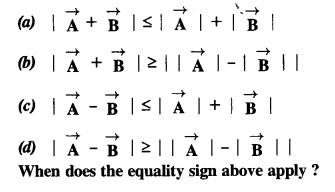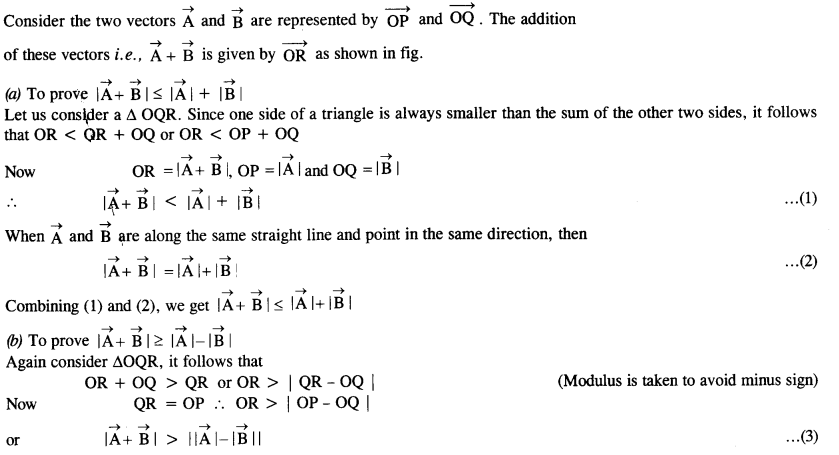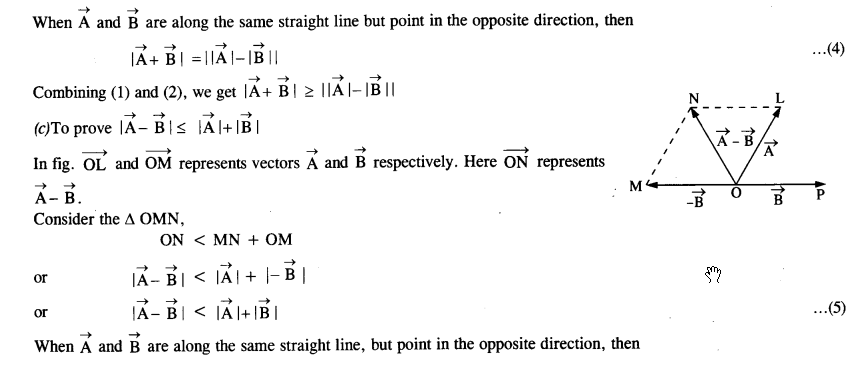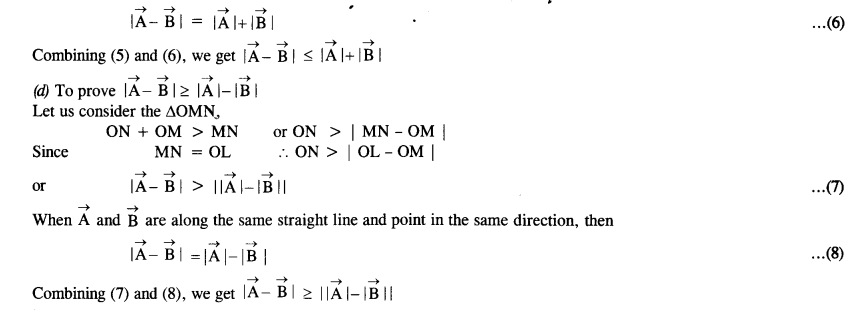Question 7.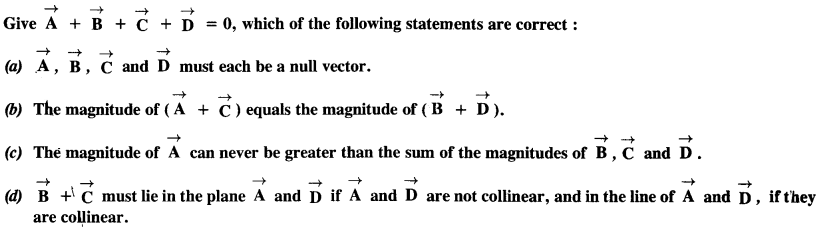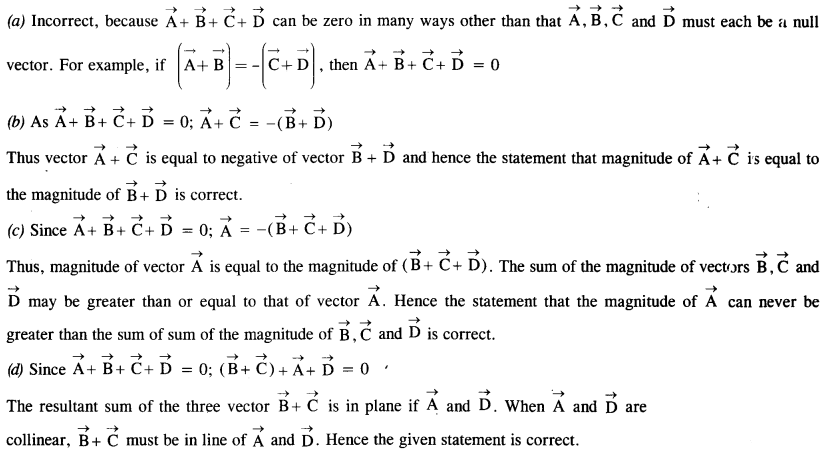Question 8.
Three girls skating on a circular ice ground of radius 200 m start from a point P on the edge of the ground and reach a point Q diametrically opposite to P following different paths as shown in Fig. What is the magnitude of the displacement vector for each ? For which girl is this equal to the actual length of path skate ?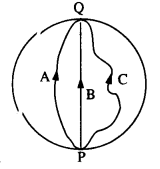(1) PQ = diameter = 2r = 2 x 200 = 400 m
Since displacement vector does not depend upon the actual path length and it is the shortest distance between initial and final position, so in the case of each girl the displacement is 400 m.
(2) Actual path length in the case of girl B is least e., equal to the displacement vector.

Question 9.
A cyclist starts from the center O of a circular park of radius 1 km, reaches the edge P of the park, then cycles along the circumference, and returns to the center along QO as shown in Fig. If the round trip takes 10 min, what is the
(a) net displacement,
(b) average velocity, and
(c) the average speed of the cyclist?Question 10.
On open ground, a motorist follows a track that turns to his left by an angle of 60° after every 500 m. Starting from a given turn, specify the displacement of the motorist at the third, sixth and eighth turn. Compare the magnitude of the displacement with the total path length covered by the motorist in each case.
(1) The path Followed by the motorist will be a closed hexagonal path.
Suppose the motorist starts his journey from point O. He takes the turn at point C.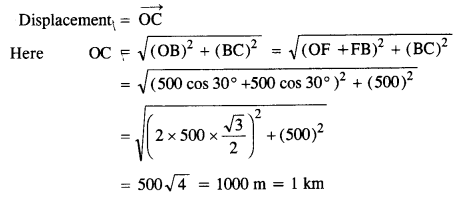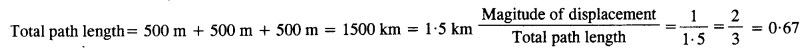(2) The motorist will take the sixth turn at O.
Displacement is zero Path length is 3000 m i.e., 3 km
Ratio of magnitude of displacement and path length is zero.

(3) The motorist will take the 8th turn at B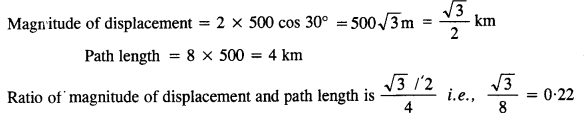Question 11.
A passenger arriving in a new town wishes to go from the station to a hotel located 10 km away on a straight road from the station. A dishonest cabman takes him along a circuitous path 23 km long and reaches the hotel in 28 min. What is
(a) the average speed of the taxi,
(b) the magnitude of average velocity? Are the two equal?
Magnitude of displacement = 10 km
Total path length = 23 km
Time taken=28 min=$$\cfrac { 28 }{ 60 }$$= $$\cfrac { 7 }{ 15 }$$ h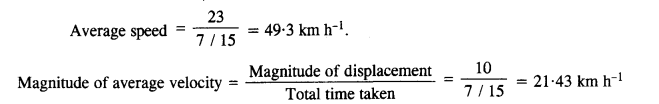Question 12.
Rain is falling vertically with a speed of 30 ms-1. A woman rides a bicycle with a speed of 10 ms-1 in the north to south direction. What is the direction in which she should hold her umbrella?In order to protect herself from rain, the woman should hold her umbrella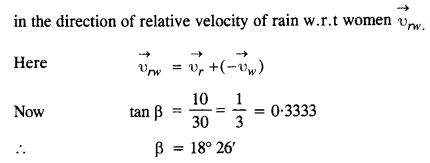So a woman should hold her umbrella at an angle of 18°26′ with the vertical, towards the south.

Question 13.

A man can swim at a speed of 4 km/h in still water. How long does he take to cross a river 1km wide if the river flows steadily at 3 km/h and he makes his strokes normal to the river current? How far down the river does he go when he reaches the other bank?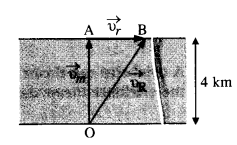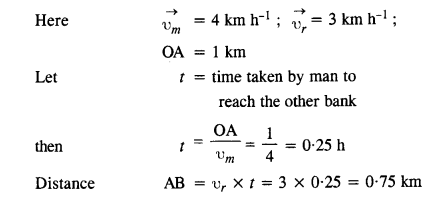Question 14.
In harbour, the wind is blowing at the speed of 72 km/h, and the flag on the mast of a boat anchored in the harbour along the N-E direction. If the boat starts moving at a speed of 51 km/h to the north, what is the direction of the flag on the mast of the boat?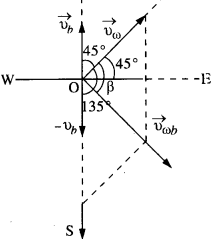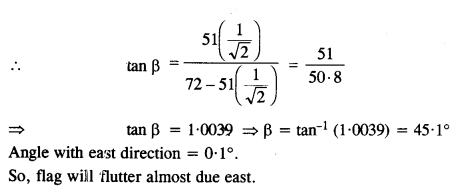Question 15.
The ceiling of a long hall is 25 m high. What is the maximum horizontal distance that a ball thrown with a speed of 40 ms-1 can go without hitting the ceiling of the hall?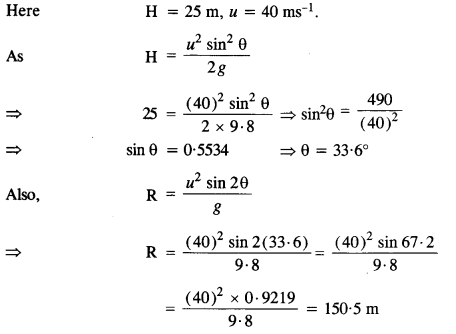Question 16.
A cricketer can throw a ball to a maximum horizontal distance of 100 m. How much high above the ground can the cricketer throw the same ball?Question 17.
A stone tied to the end of a string 80 cm long is whirled in a horizontal circle with a constant speed. If the stone makes 14 revolutions in 25 s, what is the magnitude and direction of acceleration of the
stone ?Question 18.
An aircraft executes a horizontal loop of radius 1 km with a steady speed of 900 km/h. Compare its centripetal acceleration with the acceleration due to gravity.
Here r = 1 km = 103 m, υ= 900 km h_1 = 900 x $$\cfrac { 5 }{ 18 }$$= 250 ms_1.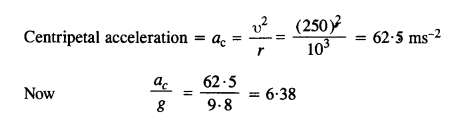Question 19.
Read each statement below carefully and state, with reasons, if it is true or false :
(a) The net acceleration of a particle in circular motion is always along the radius of the circle towards the center.
(b) The velocity vector of a particle at a point is always along the tangent to the path of the particle at that point
(c) The acceleration vector of a particle in uniform circular motion averaged over one cycle is a null vector.
(a) False, the net acceleration of a particle in circular motion is along the radius of the circle towards the center only in uniform circular motion.
(b) True
(c) True. (The direction of acceleration vector of a particle in uniform circular motion changes continuously. The sum of the vectors represented by a closed polygon taken in the same order is zero.)

Question 20.
The position of a particle is given by
$$\vec { r }$$=3.0 t $$\hat { i }$$-2.0 t2 $$\hat { i }$$+4.0$$\hat { k }$$ m where t is in seconds and the coefficients have the proper units for r to be in meters.
(a) Find the v and a of the particle,
(b) What is the magnitude and direction of velocity of the particle at t = 3s?Question 21.
A particle starts from the origin at t = 0 with a velocity of 10.0 $$\hat { j }$$ m/s and moves in the x-y plane with a constant acceleration of (8.0 $$\hat { i }$$ +2.0  $$\hat { j }$$)
ms-1
(a) At what time is the x-coordinate of the particle 16 m ? What is the y- coordinate of the particle at that time ?
(b) What is the speed of the particle at the time ?
(a) The position of the particle is given by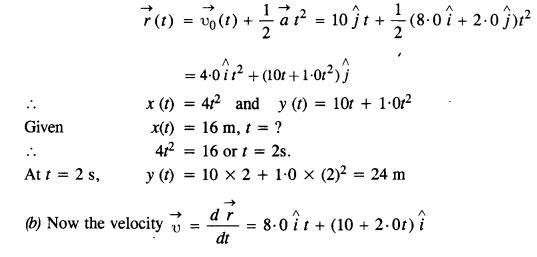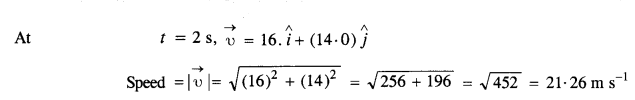Question 22.
$$\hat { i }$$ and $$\hat { j }$$ are unit vectors along x-and y-axis respectively. What is the magnitude and direction of the vectors. $$\hat { i }+ { j }$$ and $$\hat { i }-{ j }$$ ? What are the components of a vector $$\vec { A }=$$ $$\hat { 2i }+ { 3j }$$ along the directions of $$\hat { i }+ { j }$$ and  $$\hat { i }-{ j }$$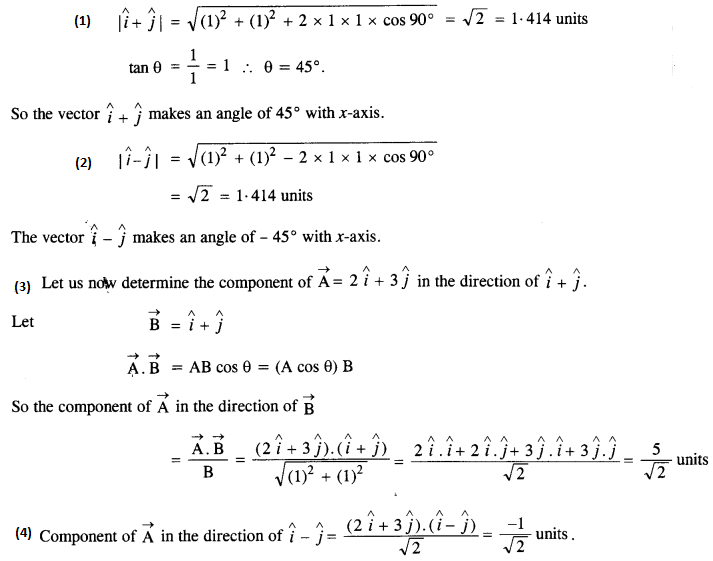Question 23.
For any arbitrary motion in space, which of the following relations are true:
(a) υaverage (1/2) (υ (t1+υ t2)
(b) υaverage = [r (t1) – r (t2)]-(t2-t1)
(c) υ(t) = υ(0) + at
(d) r(t) = r(0) + υ(0) t + (1/2) at2
(e) υaverage =υ(t2) – υ (t1)]/(t2 – t1)
(The ‘average’ stands for an average of the quantity over the time interval t1 to t2)
(b) and (e) is true. For arbitrary motion, acceleration may not be constant. Hence, (a), (c) and (d) are not true.

Question 24.
Read each statement below carefully and state, with reasons and examples, if it is true or false :
A scalar quantity is one that

1. is conserved in a process
2. can never take a negative value
3. must be dimensionless
4. does not vary from one point to another in space
5. has the same value for observers with different orientations of axes.

1. False, e.g. kinetic energy is a scalar quantity. In inelastic collisions, kinetic energy does not remain conserved.
2. False, e.g. temperature is a scalar quantity. It can be positive as well as negative on the Celsius scale.
3. False, e.g. mass is a scalar quantity but it is not dimensionless.
4. False, e.g. gravitational potential is a scalar and it varies from one point to another in the space.
5. True, A mass m kg will remain m kg for observers with different orientations of axes.

Question 25.
An aircraft is flying at a height of 3400 m above the ground. If the angle subtended at a ground observation point by the aircraft positions 10 s apart is 30°, what is the speed of the aircraft?
Let A and B be the two positions of the aircraft separated by 10 s, then from the figure.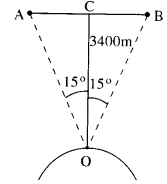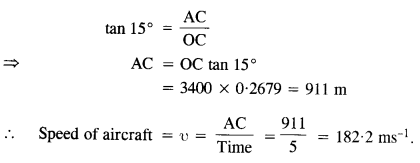Question 26.
A vector has magnitude and direction. Does it have a location in space? Can it vary with time? Will two equal vectors’ $$\vec { A } and { B }$$ at different locations in space necessarily have identical physical effects ? Give examples in support of your answer.
A vector does not have any location in space. No, it can not vary with time provided its magnitude and direction are given. For Example, $$\vec { A }=$$ $$\hat {2i }+{ 2j }+{ 5j }$$ does not vary with time. Two equal vectors at different locations in space may have identical physical effects. For example, two bodies fall freely with the same acceleration at two positions if acceleration due to gravity at these two positions is equal.

Question 27.
A vector has both magnitude and direction. Does it mean that anything that has magnitude and direction is necessarily a vector? The rotation of a body can be specified by the direction of the axis of rotation, and the angle of rotation about the axis. Does that make any rotation a vector?
No. Anything that has magnitude and direction is a vector only if it obeys the laws of vector addition. The finite rotation of a body about an axis is not a vector because finite rotation does not obey the commutation law.

Question 28.
Can you associate vectors with

1. the length of a wire bent into a loop
2. a plane area
3. a sphere? Explain.

1. Since direction at every point on the loop changes, so vector cannot be associated with the length of a wire bent in a loop.
2. Vector can be associated with the plane area. Such a vector is called an area vector and its direction is taken as normal to the plane.
3. Since the direction of a perpendicular drawn at every point on a sphere is different, so a vector cannot be associated with a sphere.

Question 29.
A bullet fired at an angle of 30° with the horizontal hits the ground 3 km away. By adjusting its angle of projection, can one hope to hit a target 5 km away? Assume the muzzle speed to the fixed, and neglect air resistance.
Here R = 3 km = 3000 m, θ = 30°, g = 9.8 m s-2.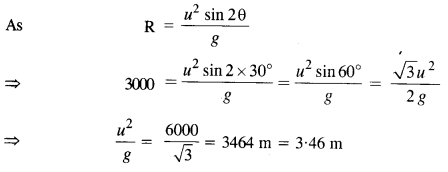Maximum range of bullet =u2/g=3-46 m. Since the distance of the target (5 km) is greater than the maximum range of

Question 30.
A fighter plane flying horizontally at an altitude of 1.5 km with a speed of 720 km h-1 passes directly overhead an anti-craft gun. At what angle from the vertical should the gun be fired for the shell muzzle speed 600 m s-1  to hit the plane? At what maximum altitude should the pilot fly the plane to avoid being hit? Take g = 10 m s-2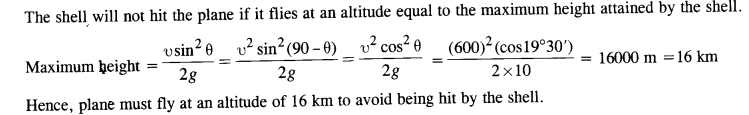Question 31.
A cyclist is riding at a speed of 27 km/h. As he approaches a circular turn on the road of a radius of 80 m, he applies brakes and reduces his speed at the constant rate of 0.5 m/s every second. What is the magnitude and direction of the net acceleration of the cyclist on the circular turn?Question 32.
(a) Show that for a projectile, the angle between the velocity and the x-axis as a function of time is given by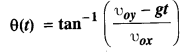(b) Show that the projection angle θo for a projectile launched from the origin is given by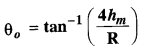where the symbols have their usual meaning.